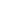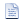A Survey of Gaussian Convolution Algorithms
File List
Here is a list of all documented files with brief descriptions:basic.c Portable types, math constants, and timingbasic.h Portable types, math constants, and timingcomplex_arith.h Complex arithmeticerfc_cody.c W.J. Cody's approximation of the complementary error function (erfc)erfc_cody.h W.J. Cody's approximation of the complementary error function (erfc)filter_util.c Filtering utility functionsfilter_util.h Filtering utility functionsgaussian_bench.c Benchmark for testing Gaussian convolution algorithmsgaussian_conv_am.c Alvarez-Mazorra approximate Gaussian convolutiongaussian_conv_am.h Alvarez-Mazorra approximate Gaussian convolutiongaussian_conv_box.c Box filtering approximation of Gaussian convolutiongaussian_conv_box.h Box filtering approximation of Gaussian convolutiongaussian_conv_dct.c Gaussian convolution via DCTgaussian_conv_dct.h Gaussian convolution via DCTgaussian_conv_deriche.c Deriche's approximation of Gaussian convolutiongaussian_conv_deriche.h Deriche's approximation of Gaussian convolutiongaussian_conv_ebox.c Gaussian convolution with extended box filtersgaussian_conv_ebox.h Extended box filter Gaussian approximationgaussian_conv_fir.c Gaussian convolution using FIR filtersgaussian_conv_fir.h Gaussian convolution using FIR filtersgaussian_conv_sii.c Gaussian convolution using stacked integral imagesgaussian_conv_sii.h Gaussian convolution using stacked integral imagesgaussian_conv_vyv.c Vliet-Young-Verbeek approximate Gaussian convolutiongaussian_conv_vyv.h Vliet-Young-Verbeek approximate Gaussian convolutiongaussian_demo.c Gaussian convolution demogaussian_short_conv.c Gaussian convolution for short signals (N <= 4)gaussian_short_conv.h Gaussian convolution for short signals (N <= 4)imageio.c Implements read_image and write_image functionsimageio.h Implements read_image and write_image functionsimdiff.c Image difference calculator programinverfc_acklam.c Acklam's algorithm for the inverse complementary error functioninverfc_acklam.h Acklam's algorithm for the inverse complementary error functioninvert_matrix.c Invert matrix through QR decompositioninvert_matrix.h Invert matrix through QR decompositionnum.h Num typedefstrategy_gaussian_conv.c Suite of different 1D Gaussian convolution methodsstrategy_gaussian_conv.h Suite of different 1D Gaussian convolution methods